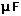# RC Low Pass Filter

Since capacitive reactance decreases with frequency, the RC circuit shown discriminates against high frequencies. The circuit is an AC voltage divider with an output which falls off at high frequencies at the rate of 6 dB per octave.CalculationMore detail
 Filter Circuits
Index

Capacitance concepts

Inductance concepts

 HyperPhysics*****Electricity and magnetism R Nave
Go Back

# RC Low Pass Filter DetailsRC low pass filter Calculation
 Filter Circuits
Index

Capacitance concepts

Inductance concepts

 HyperPhysics*****Electricity and magnetism R Nave
Go Back

# RC Low Pass Filter Calculation

Since capacitive reactance decreases with frequency, the RC circuit shown discriminates against high frequencies. The circuit is an AC voltage divider with an output which falls off at high frequencies at the rate of 6 dB per octave.More detail

One approach to the design of a low-pass filter is to establish a frequency "breakpoint" or "knee" by choice of the resistance and capacitance. The design calculation works by clicking on the desired quantity in the expression. Enter the necessary data and then click on the quantity you wish to calculate. Default values will be entered for unspecified quantities, but all quantities may be changed. After the filter has been designed, the amount of attenuation in decibels at any frequency can be evaluated by the separate calculation below. Once you get well above the breakpoint frequency, the amount of attenuation will increase by about 6dB per octave, which you can confirm with that exploratory calculation.

Breakpoint = 1/(ResistancexCapacitance)

 For R = x10^ Ω = kΩ = MΩ
 and C = x10^ F == pF
 the breakpoint angular frequency ω = x10^ rad/s
and frequency = x10^ Hz = kHz = MHz

and the capacitive reactance at the breakpoint is

 XC = x10^ ohms = kohms = Megohms

Evaluation of filter action:
 If the input angular frequency ωin = x10^ rad/s
 the output ratio Vout/Vin = x10^ = dB.

 Filter Circuits
Index

Capacitance concepts

Inductance concepts

 HyperPhysics*****Electricity and magnetism R Nave
Go Back